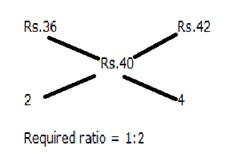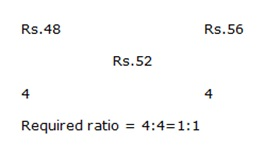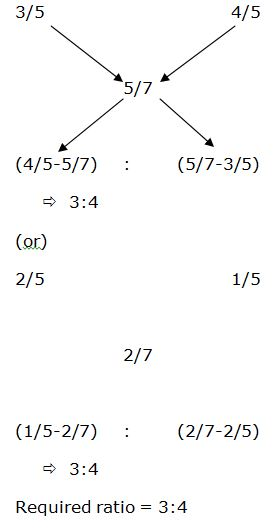# Quantitative Aptitude Questions (Mixture and Alligation) for SBI Clerk 2018 Day-25

## Quantitative Aptitude Questions (Mixture and Alligation) for SBI Clerk 2018 Day-25:

Dear Readers, SBI is conducting Online preliminary Examination for the recruitment of Clerical Cadre. preliminary Examination of SBI Clerk was scheduled from March 2018. To enrich your preparation here we have providing new series of Mixture and Alligation – Quantitative Aptitude Questions. Candidates those who are appearing in SBI Clerk Prelims Exam can practice these Quantitative Aptitude average questions daily and make your preparation effective.

[WpProQuiz 1359]

Click “Start Quiz” to attend these Questions and view Solutions

## Daily Practice Test Schedule | Good Luck

 Topic Daily Publishing Time Daily News Papers & Editorials 8.00 AM Current Affairs Quiz 9.00 AM Logical Reasoning 10.00 AM Quantitative Aptitude “20-20” 11.00 AM Vocabulary (Based on The Hindu) 12.00 PM Static GK Quiz 1.00 PM English Language “20-20” 2.00 PM Banking Awareness Quiz 3.00 PM Reasoning Puzzles & Seating 4.00 PM Daily Current Affairs Updates 5.00 PM Data Interpretation / Application Sums (Topic Wise) 6.00 PM Reasoning Ability “20-20” 7.00 PM English Language (New Pattern Questions) 8.00 PM General / Financial Awareness Quiz 9.00 PM

Q.1) A vessel contains milk and water in which 20% water. 20 litres of mixture was taken out and replaced by water and the ratio becomes 12:13. Find the initial quantity of milk in the vessel

1. 40 litres
2. 30 litres
3. 50 litres
4. 56 litres
5. 60 litres

Q.2) A container contains pure milk. From these 4 litres of milk taken out and replaced by water. This process is repeated for one more time and the remaining milk in the container is 12.8 litres. What is the initial quantity of milk in the container?

1. 15 litres
2. 20 litres
3. 25 litres
4. 24 litres
5. 32 litres

Q.3) A trader mixes two types of rice varieties with a cost of Rs.36 and Rs.42. If he sells the mixture of Rs.44 at 10% profit, in what ratio he mixes two types of rice varieties?

1. 1:2
2. 1:3
3. 2:3
4. 2:5
5. 3:2

Q.4) How many kg of rice variety 1 costing Rs.48/kg should a shopkeeper mix with 20 kg of rice variety 2 costing Rs.56 per kg so that he makes a profit of 20% on selling the mixture at Rs.62.4/kg?

1. 10 kg
2. 15 kg
3. 12 kg
4. 20 kg
5. 24 kg

Q.5) How many litres of water should be added to a 60 litres mixture containing milk and water in the ratio of 2:1 such that the resultant mixture has 50% milk in it?

1. 15 litres
2. 20 litres
3. 30 litres
4. 10 litres
5. 25 litres

Q.6) A vessel contains 40 litres of pure wine. Find the amount of water mixing if he sells the mixture at cost price and gains 20%

1. 12 litres
2. 24 litres
3. 10 litres
4. 15 litres
5. None of these

Q.7) A bucket contains 60 litres of pure wine, in which x litres drawn off and replaced with water. This process is repeated for two times. Find the value of x if the final ratio of wine to water is 81:19

1. 4 litres
2. 6 litres
3. 2 litres
4. 5 litres
5. 10 litres

Q.8) Two equal vessels A and B contain 60% of sugar and 40% of sugar respectively and the remaining Rava. In which 40 kg of mixture is taken out from vessel A and replaced into vessel B. Find the initial quantity of vessel if the final ratio of sugar and Rava in vessel B is 16:19

1. 120 litres
2. 150 litres
3. 80 litres
4. 100 litres
5. 60 litres

Q.9) A bucket contains some quantity of milk and water, in the ratio of water and milk is 3:5. 40 litres of mixture is drawn out and replaced with water and the ratio of milk and water becomes 5:11 then find the initial quantity of milk

1. 40 litres
2. 50 litres
3. 25 litres
4. 30 litres
5. 45 litres

Q.10) There are two vessels A and B contains wine and water in the ratio of 3:2 and 4:1 respectively. In what ratio of wine and water should be mixed if the ratio of wine and water is 5:2

2:5

7:9

1:2

3:7

None of these

Explanation:

Milk and water ratio = 4:1

Given,

(4x-16)/(x-4+20) =12/13

13x-52 = 3x+48

=>10x=100=>x=10

Initial quantity of milk in the vessel= 40 litres

Let us take initial quantity of a container be x

Remaining milk = Initial (1-Replaced/Initial)n

12.8= x (1-4/x)2

12.8x=x2+16-8x

5X2-104x+80=0

Simplify the above equation, we get x= 20 and 0.8 (Eliminate)

CP of Mixture = 44/110*100=Rs.40CP of mixture = 62.4/120*100=Rs.52Required kg = 20/1*1=20 kg

Total mixture=60 litres

Milk and water in the ratio of 2:1,

So, Milk=40 lit, water=20 lit

Given,

40/(20+x)=1/1

40=20+x=>x=20 litres

He sells the mixture at cost price and gains 20%. Here gain will be the water. So,

80% of mixture =40 litres

=>Mixture=40/80*100=50 litres

Amount of water added = 50-10=10 litres

Given,

Wine and water in final process (60/100*81=48.6) and (60/100*19=11.4) respectively

48.6=60 (1-x/60)2

48.6/60=(1-x/60)2

81/100 = (1-x/60)2

9/10=1-x/60

X/60=1-9/10=>x/60=1/10=>x=6 litres

Vessel A Sugar and Rava ratio = 3:2

Vessel B sugar and Rava ratio = 2:3

Given,

(2x+24)/(3x+16)=16/19

38x+456=48x+256

10x=200=>x=20 litres

Initial quantity = x*5=100 litres

(5x-25)/(3x-15+40)=5/11

55x-275=15x+125

=>x=10 litres

Initial quantity of milk = 10*5=50 litres